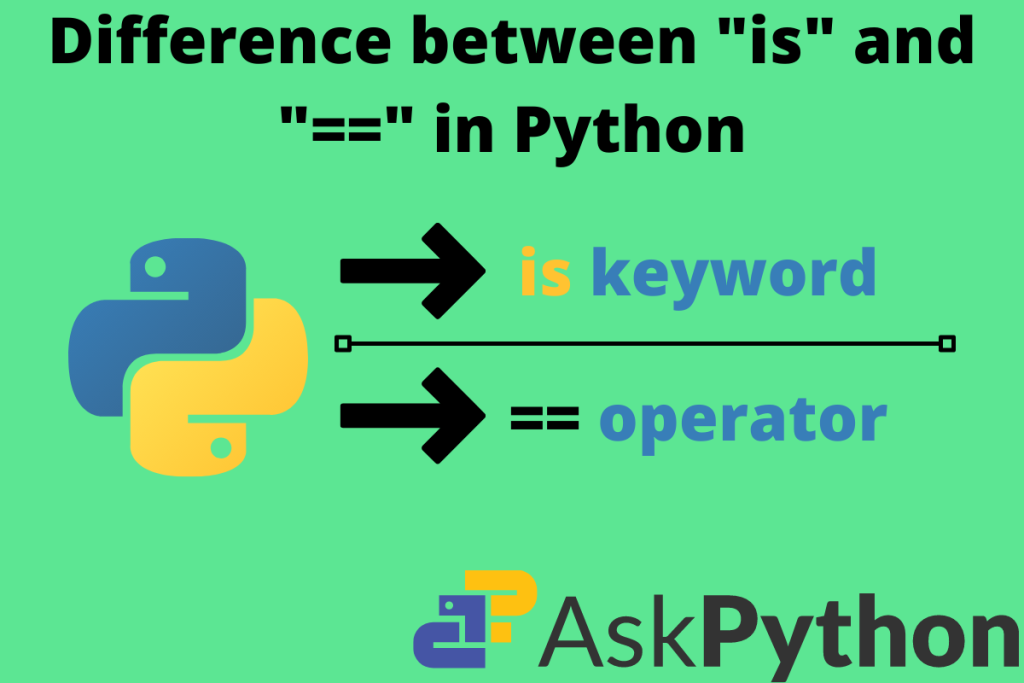# Difference between “is” and “==” in PythonHey fellas!, as we all know that Python has some of the benefits that most interpreted languages do not give. Some of them are the flexibility concepts that we use to perform when doing mathematical calculations. While doing so, we get some confusion about the operators and keywords. Now, in this article, our aim is to get the difference between the same. The is and double equal-to operator. So, let’s get into it.

## What are operators in Python?

Operators in any programming language are the main fundamental concepts behind the basics. There are the following operators in Python:

1. Logical: to perform the logical calculations
1. and
2. or
3. not
2. Arithmetic: to perform basic arithmetic calculations
2. : multiplication
3. / : division
4. % : modulo (returns the remainder)
5. // : floor division operator (returns integer value for float division)
3. Exponential: calculating the power and XOR value of numbers
1. ** : power
2. ^ : XOR

## Let’s code the concepts and trace the difference

Now, here we will compare the keywords and try to trace the difference in their operation.

### The “==” operator

Let us take the example of two variables. The variables are having different values each. Say a has 20 and b has 30. Now, we all know that they are not equal. But, how the computer shall recognize this? for this purpose only we have the double equal-to operator. Let us take a code example of this:

Code:

```a = 30
b = 30
if a == b:
print(True)
else:
print(False)
```

Output:

```True
```

Here we can state that the main function of equal to is to check whether the values are the same or not. For a complex example we can also check with functions:

Code to check the square root of a number:

```from math import sqrt
def square_root(val):
return (val**(1/2))

a = sqrt(9)
b = square_root(9)
print(a == b)
```

Output:

```True
```

Here we compare the value returned by the two functions. The values they return are the same but, the identification of both in the interpreter is different.

```print(id(a))
print(id(b))
```

Output:

```2178644181968
2178644086384
```

Here the double equal to operator shows a different nature. It shall return false when we compare their identities. It returns false, so to tackle this we use the “is” operator.

### The is keyword

This keyword is for the comparison of values as well as for object reference. When we create the object of any class it is the instance that holds the properties of each class. For every new object created the interpreter assigns a new identity to each one of them.

#### Example with numbers

```a = 10
b = 10.0
print(a is b)
```

Output:

```False
```

As we can see that both are 10 but one is a float and another is an integer. So, let the values look similar, the object type can be different

#### Example with data structures

In this example, we have two lists a and b. They both contain the same values of elements. But, when we try to compare them using the “is” keyword the output is surprising.

```a = [1, 2, 3, 4, 5]
b = [1, 2, 3, 4, 5]

print(type(a))
print(type(b))

if (a is b):
print("Successful")

else:
print("Unsuccessful")
```

Output:

```<class 'list'>
<class 'list'>
False
```

Explanation:

1. Both lists have the same class as <class “list”>.
2. But, the main issue is that the memory block assigned to l1 is different from that of l1.
3. The is operator checks the memory location of the object that we create.
4. The allocation of blocks of memory is different for every object. This makes the is return False value.

#### For example with a NumPy array

Code:

```import numpy as np
a = np.array([[1, 2], [3, 4]])
b = np.array([[1, 2], [3, 4]])
print(a is b)
```

Output:

```False
```

The same goes for a NumPy array. Both the arrays are the same, but, the memory allocated to them is different. Their id is different. So, we get False.

#### For example with a class

```class temp_1():
def __init__(self, a, b):
self.a = a
self.b = b

def print_val(self):
print(self.a, self.b)

class temp_2():
def __init__(self, a, b):
self.a = a
self.b = b

def print_val(self):
print(self.a, self.b)

obj1 = temp_1(30, 40)
obj2 = temp_2(30, 40)
obj1.print_val()
obj2.print_val()

print((obj1 is obj2))
```

Output:

```30 40
30 40
False
```

Explanation:

Both classes have the same properties, same functions as well as same parameters. The notable difference is that they are not the same by memory reference. So, the conclusion through this code experiment is: For more efficient testability of code is keyword is more useful than the == operator.

## Summary

The double is equal to check only for value but, check for both value and reference. So, this article narrates to us the difference between “is” and “==” in Python and how we can efficiently use both the concepts in Python programming and make our code more robust.